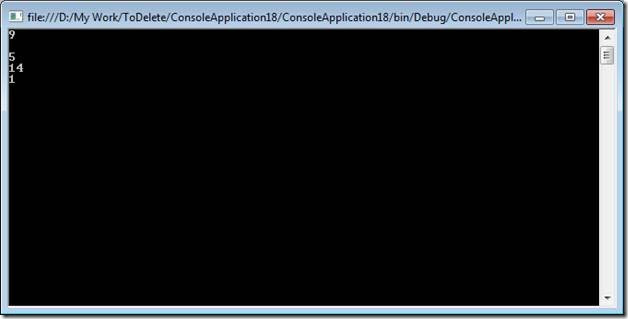# Delegate to Lambda Expression

Objective
This article will not give any theoretical definition of
1. Delegate Read theory of Delegate here
2. Anonymous method
3. Lambda Expression Read theory of Lambda expression here
I am going to give a story type discussion from Delegate to Lambda expression.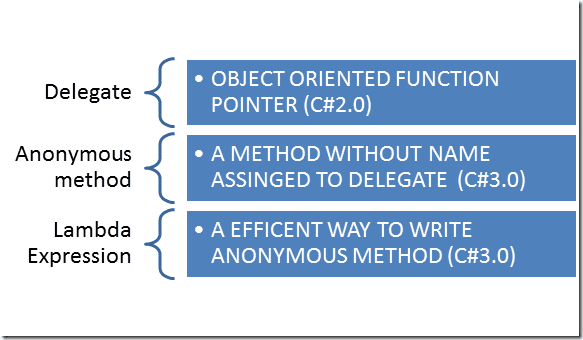Let us say, there is a requirement that you need to pass one function as parameter of another function. This can be easily done through function pointer in language like C++ but in C#, we cannot pass a function.
There are two functions
``` ```

`    int Add(int number1, int number2)`
`        {`
`            return number1 + number2;`
`        }`
`     int Sub(int number1, int number2)`
`        {`
`            return number1 - number2;`
`        }`
Now we need to pass the above function in other function called Display.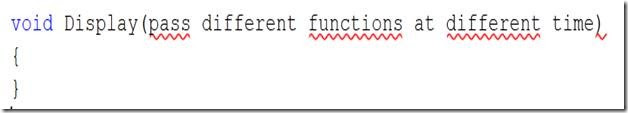Sometime we need to pass add function or sometime we need to pass Sub function to display the result of the add or sub.
So here in our requirement we will have to pass something, which can represent both Add and Sub function.
If we notice, signature of both Add and Sub function is exactly the same. Both are returning an Integer parameter and taking as input two integer parameters.
So if we can have something which can represent Add and Sub function then we can pass that representation as parameter.
So here delegate comes into picture. We will pass delegate of Add and Sub function as parameter of Display function.
Now signature of Display function can be modified as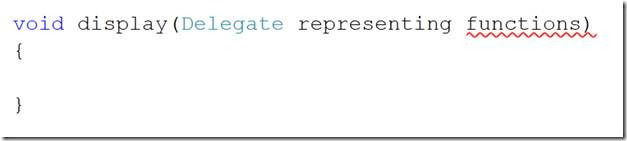So now we have the task to create the delegate. We can say delegate is representation of function. While creating a delegate only one thing we need to keep in minds that, “Delegate can refer only those functions which are having exactly the same signature as of delegate “
So for our purpose we will have to declare a delegate with return type integer and taking two integer parameters.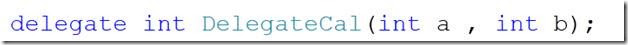In above delegate declaration
1. Integer is return type.
2. Name of the delegate is DelegateCal
3. There are two integer input parameter.
So, DelegateCal only can refer functions which are having integer return type and two integer parameters.
So now we can assign a function to delegate as below,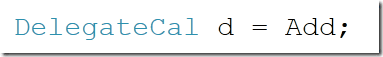Here signature of Add and DelegateCal is same. Not a point here is that, you can assign function with different signature to delegate else you will be get a compile time error.
So, now Display function can be modified as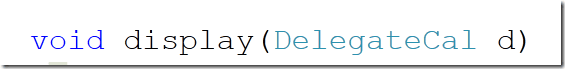Now we are meeting our requirement and we can pass any function with the signature matching of the delegate to the display function.
Program.cs
``` ```

`using System;`
`using System.Collections.Generic;`
`using System.Linq;`
`using System.Text;`
`namespace ConsoleApplication18`
`{`
`    class Program`
`    {`
`        delegate int DelegateCal(int a , int b);`
`        static void Main(string[] args)`
`        {`
`            DelegateCal d = Add;`
`            display(d);`
`            Console.Read();`
`            d = Sub;`
`            display(d);`
`            Console.ReadKey(true);`
`        }`
`      static  void display(DelegateCal d)`
`        {`
`          int result = d(7,2);`
`          Console.WriteLine(result);`
`        }`
`      static int Add(int number1, int number2)`
`        {`
`            return number1 + number2;`
`        }`
`     static int Sub(int number1, int number2)`
`        {`
`            return number1 - number2;`
`        }`
`    }`
`}`
Explanation
1. We have declared a delegate with signature, return type integer and two integer input parameters.
2. Created two functions Add and Sub. Both are taking two integer parameters and returning an integer.
3. Created a Display function which is taking delegate as parameter.
4. Assigning the function to the delegate.
5. Calling the display function.
Output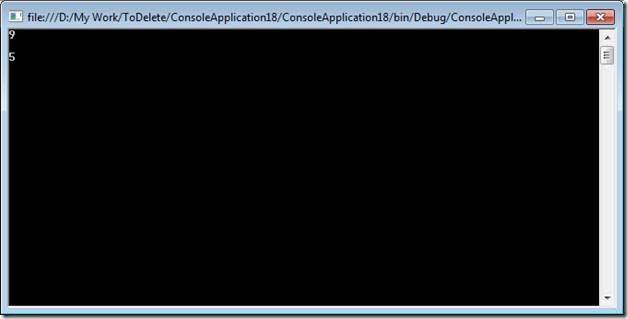Anonymous method
Now let us say you need to create a function for multiply. Now we have an option that we can create a function for multiply [with signature same as delegate]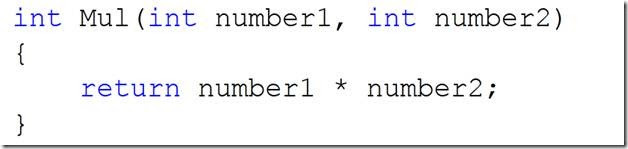But, what if we don’t want to create a function rather we want the function at the run time or on the fly without any name. We can very much achieve that using anonymous method.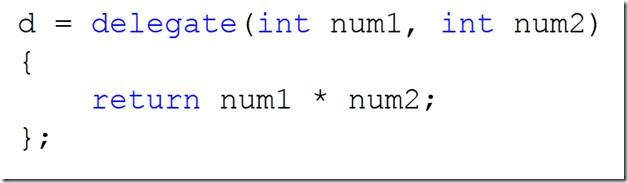In above code,
1. d is the delegate.
2. delegate keyword is being used to create an anonymous method.
3. Anonymous method does not have any name
4. There are two input parameters for anonymous method.
5. d now can be passed as parameter to display function.
So from above code we can conclude that we have created a function without name (anonymous method) and assigned that to a delegate with the same signature.
So now rather than creating a function explicitly using anonymous method we are creating that on the fly or at the run time.
Program.cs
``` ```

`using System;`
`using System.Collections.Generic;`
`using System.Linq;`
`using System.Text;`
`namespace ConsoleApplication18`
`{`
`    class Program`
`    {`
`        delegate int DelegateCal(int a , int b);`
`        static void Main(string[] args)`
`        {`
`            DelegateCal d = Add;`
`            display(d);`
`            Console.Read();`
`            d = Sub;`
`            display(d);`
`            Console.ReadKey(true);`
`            d = delegate(int num1, int num2)`
`            {`
`                return num1 * num2;`
`            };`
`            display(d);`
`            Console.ReadKey(true);`
`        }`
`      static  void display(DelegateCal d)`
`        {`
`          int result = d(7,2);`
`          Console.WriteLine(result);`
`        }`
`      static int Add(int number1, int number2)`
`        {`
`            return number1 + number2;`
`        }`
`     static int Sub(int number1, int number2)`
`        {`
`            return number1 - number2;`
`        }`
`    }`
`}`
Output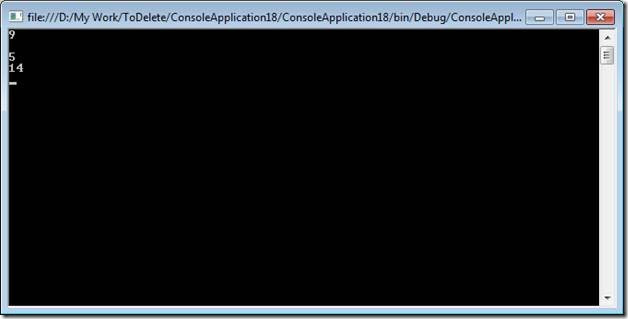Lambda expression
If we see the anonymous method
1. We are using the keyword delegate.
2. We are explicitly giving the data type of input variables.
3. Readability is not very high.
Lambda expression allows us to write anonymous function in very concise way. Readability is high.
Lambda expression uses goes to operator.
So, if we modify the above anonymous method as below,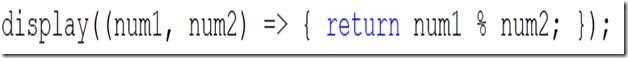In above code we are directly in lining the code for division, rather than creating any function or anonymous method.
Program.cs
``` ```

`using System;`
`using System.Collections.Generic;`
`using System.Linq;`
`using System.Text;`
`namespace ConsoleApplication18`
`{`
`    class Program`
`    {`
`        delegate int DelegateCal(int a , int b);`
`        static void Main(string[] args)`
`        {`
`            DelegateCal d = Add;`
`            display(d);`
`            Console.Read();`
`            d = Sub;`
`            display(d);`
`            Console.ReadKey(true);`
`            d = delegate(int num1, int num2)`
`            {`
`                return num1 * num2;`
`            };`
`            display(d);`
`            Console.ReadKey(true);`
`            display((num1, num2) => { return num1 % num2; });`
`            Console.ReadKey(true);`
`        }`
`      static  void display(DelegateCal d)`
`        {`
`          int result = d(7,2);`
`          Console.WriteLine(result);`
`        }`
`      static int Add(int number1, int number2)`
`        {`
`            return number1 + number2;`
`        }`
`     static int Sub(int number1, int number2)`
`        {`
`            return number1 - number2;`
`        }`
`    }`
`}`
Output